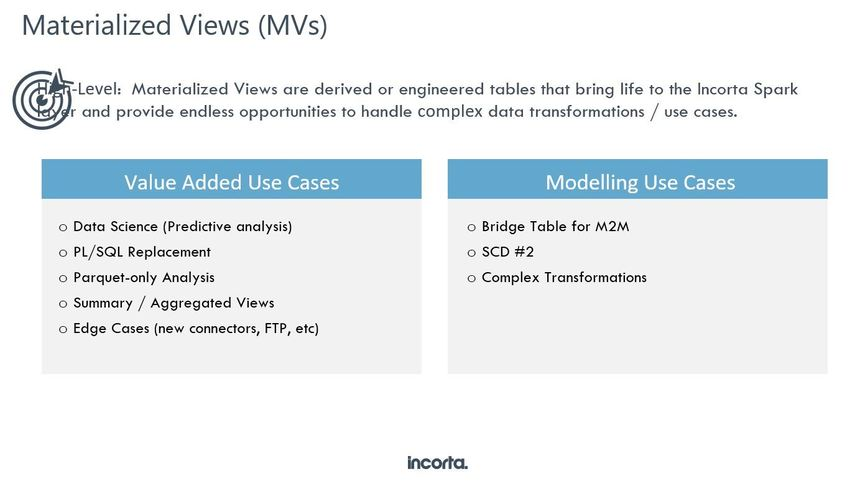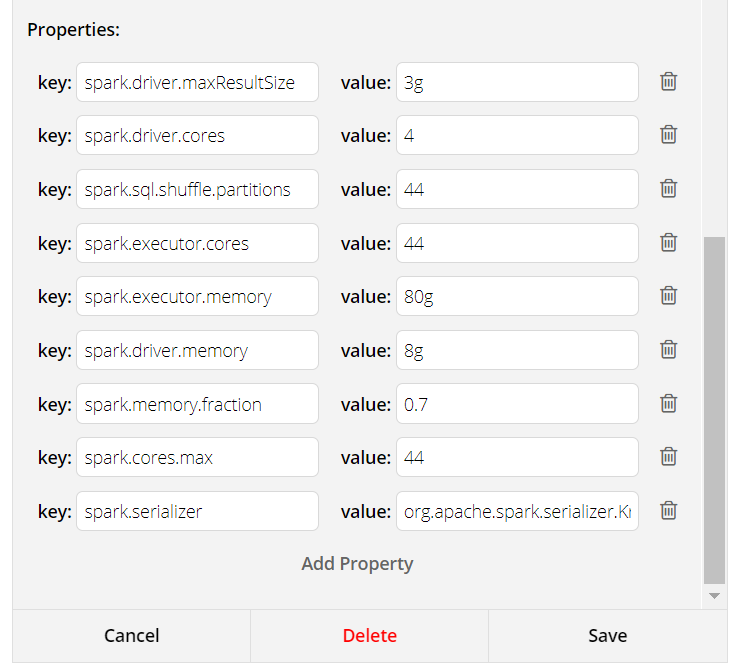13

# Creating Materialized View using PySpark

Created by Amit Kothari

Incorta allows you to create Materialized Views using Python and Spark to read the data from the Parquet files of existing Incorta Tables, transform it and persist the data so that it can be used in Dashboards.We can leverage the power of scripting via Python and Spark to handle  many usecases , for eg -

• Creating bridge tables to resolve many to many relations .
• Creating an aggregate table from a detail table and using that to join as a parent table - for eg let us say we have a line table and a distribution table which is at a lower grain than the line table. Now we want to keep the dashboard query  with the line table as the base table and just want to get some aggregated metric from the distribution table. Then a MV can be created on the  distribution table with a key  of the line table which is then joined as a parent table to the line table.
• Converting Oracle PL/SQL procedures - where multi pass logic is required
• Predictive analytics using Spark ML and NumPy
• Data Quality analysis

Best Practices:

• Refer to this MV performance tuning article
• Use the language as SQL if there is only query for which you need to create a MV. For other complex usecase you can select Python as the language.
• Rather than denormalizing the dimensional attributes in the MV itself try creating a MV which has id and metric fields so that the id fields can be joined to the keys of the parent tables for resolving the dimensional attributes
• Incremental logic can be supported in a MV if a proper key can be defined on it.
• We can  use the ? inside the MV sql, ? means last successful transformation timestamp value of MV. This is similar to ? used inside our regular SQL Queries. The value is stored in a long format representing a timestamp value. Eg

Select * FROM incorta_metadata.DASHBOARD WHERE CREATIONDATE > ?

• The most accurate way to run incremental MVs is to select the max date from the MV itself to get the incremented data accurately.

Here is a simple example.

```from pyspark.sql import functions as F
s.registerTempTable("sales")

P.registerTempTable("products")

//here we are going to read the MV itself.
mv.registerTempTable("mv")

selectedSales = spark.sql("SELECT p.name, s.*  from products p inner join sales s on p.id= s.product_id
WHERE s.last_updated > (SELECT MAX(mv.last_updated) FROM mv)")

save(selectedSales)```
• In an Incremental MV please make sure that field order, field names (they are case sensitive) and field datatypes are the same in the full and incremental part of the code.  If the datatypes are not the same then use the CAST function, for eg Timestamp to Date :  CAST(the_ts AS DATE) AS the_date
• One more way to do incremental logic in a MV where we always want to refresh the last 30 days worth of data -
• ```#Filtering je_lines by creation date

from pyspark.sql.functions import lit, when, col, coalesce

from datetime import datetime, timedelta

d = datetime.today() - timedelta(days=30)
df_GL_JE_LINES = df_GL_JE_LINES.filter(df_GL_JE_LINES.EFFECTIVE_DATE > lit(d)```

Here is a sample script:

1) Shows how to create  a MV  using dataframes and joining  them via SQL , base tables are in a Incorta schema called TEST  and should have been loaded

```------------
from pyspark.sql import functions as F

df_customers.createOrReplaceTempView("customers_table")
df_submitters.createOrReplaceTempView("submitters_table")
df = spark.sql("""
SELECT DISTINCT a.submitter_id,
a.submitter_name,
a.submitter_type,
coalesce(upper(b.revenuegroupname), upper(a.customer_name)) AS submitter_finance_name,
coalesce(b.revenuegroupnumber, a.customer_agn) AS submitter_financials_customer_id,
a.vendor_id,
a.vendor_name
FROM submitters_table a
LEFT OUTER JOIN customers_table b ON a.customer_agn=b.customerid
WHERE lower(a.source_name)='test'
""")
save(df)```

------------

2.1)  Example of a Incremental MV using get_last_refresh_time()

The get_last_refresh_time() is a built-in function provided in the pyspark template to support incremental MV.  Here is an example:

```from pyspark import *
import pyspark.sql.functions as F
last_refresh_time=get_last_refresh_time()
dashboard.createOrReplaceTempView("dashboard")

df = spark.sql(
"""
SELECT  *
FROM dashboard
WHERE CREATIONDATE >  from_unixtime(%d / 1000)
""" % last_refresh_time)
save(df)

```

The last refresh time is the timestamp when the MV job was executed last time.  It is stored in the epoch time format and thus you can pass it to your Spark SQL as described above.

The assumption is that the data populated in the parquet layer has a date column that can be used to indicate how fresh the data is.  Typically, it is the last updated date column.  In the example, since we are only interested in the newly created records, we use the CREATIONDATE as the filter and compare it with last refresh time.  The CREATIONDATE has to be a column with the Timestamp data type defined in Incorta.

2.2)  Example of a Incremental MV using timedelta() ,  logic bolded below

```#incremental MV example
import pyspark.sql.functions as F
from datetime import datetime, timedelta
from pyspark.sql.functions import lit, when, col, coalesce

#Filtering by 7 days
d = datetime.today() - timedelta(days=7)
df_TRANSACTION_LINES_SOURCE = df_TRANSACTION_LINES_SOURCE.filter(df_TRANSACTION_LINES_SOURCE.DATE_MODIFIED > lit(d))

df_TRANSACTION_LINES_SOURCE.createOrReplaceTempView("TRANSACTION_LINES_SOURCE")
df_ITEMS.createOrReplaceTempView("ITEMS")

df1 = spark.sql("""
SELECT ts.ACCOUNT_ID,
ts.AMOUNT,
ts.AMOUNT_TAXED,
ts.COMPLETE_0,
CAST(ts.DATE_ARRIVE AS TIMESTAMP) + INTERVAL '8' HOUR AS DATE_ARRIVE,
COALESCE(ts.BRAND_ID, it.BRAND_ID)                AS CALCULATED_BRAND_ID
FROM TRANSACTION_LINES_SOURCE AS ts
LEFT OUTER JOIN ITEMS it
ON ts.ITEM_ID               = it.ITEM_ID
WHERE 1=1
""")

save(df1)```

3)  Example of a MV which can explode a comma separated list of values in a column:

```#Explode userString sample
from pyspark.sql import functions as F

df.createOrReplaceTempView("UserData")

df1 = spark.sql("""SELECT  c1,  userString FROM UserData  WHERE 1=1""")
df2 = df1.withColumn("User",F.explode(F.split(df1.userString, ",")))

save(df2)```

### MV Configuration

Spark configuration for a MV are listed below are based on the machine specs and impact the performance significantly. The configuration tab of a sample MV can looks like this, so start with the five mandatory properties mentioned below :Mandatory

1.      Spark.driver.memory: Amount of memory to use for the driver process, i.e. where SparkContext is initialized, in GB unless otherwise specified (e.g. 1g, 2g). This is the memory allocated for your spark logical plan and any spark actions that transfer the data to the driver, the default is 1g.

2.      Spark.executor.memory: Amount of memory to use per executor process, in GB unless otherwise specified. (e.g. 2g, 8g). This is the memory allocated for executing you logic, which the data will be loaded and transformed, default is 1g.

3.      Spark.cores.max: The maximum amount of CPU cores to request for the application from across the cluster. For example, on previous image we set the cores.max to 44, that means the MV will only use 44 cores from the cluster.

4.      Spark.executor.cores: The number of cores to use on each executor.

5.      Spark.sql.shuffle.partitions: Configures the number of partitions to use when shuffling data for joins  or aggregations, default is 200. Initially set the shuffle partition equals to cores.max or its multiple and as the data increases you can increase it by (cores.max * 2, cores.max *3, cores.max *4, …) and so on.

Note: Always use the documentation version of your running spark version.

5replies Oldest first
• Oldest first